# Elliptic Integral

(redirected from Elliptical integral)
Also found in: Dictionary.

## elliptic integral

[ə′lip·tik ′int·ə·grəl]
(mathematics)
An integral over x whose integrand is a rational function of x and the square root of p (x), where p (x) is a third- or fourth-degree polynomial without multiple roots.

## Elliptic Integral

any integral of the type

R (x, y) dx

where R(x, y) is a rational function of x and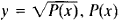being a third-or fourth-degree polynomial without multiple roots.

The integral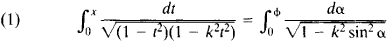is called an incomplete elliptic integral of the first kind, and the integral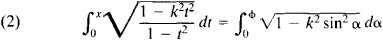is called an incomplete elliptic integral of the second kind. Here, k is the modulus of the elliptic integral, 0 < k < 1 (x = sin φ, t = sin α). The integrals on the left-hand side of equations (1) and (2) are known as Jacobi’s normal forms, and the integrals on the right-hand side are known as Legendre’s normal forms. When x = 1 or φ = π/2, the elliptic integrals are said to be complete and are designated byand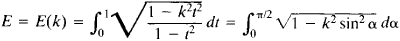respectively.

Elliptic integrals owe their name to their appearance in the problem of calculating the length of an arc of an ellipse ua sin α v = b cos α (a > b). The length of an arc of the ellipse is expressed by the formula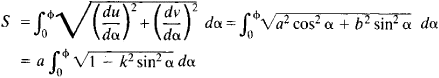where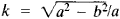is the eccentricity of the ellipse. The length of one-fourth of the circumference of an ellipse is equal to E(k). The inverse functions of elliptic integrals are called elliptic functions.

Site: Follow: Share:
Open / Close